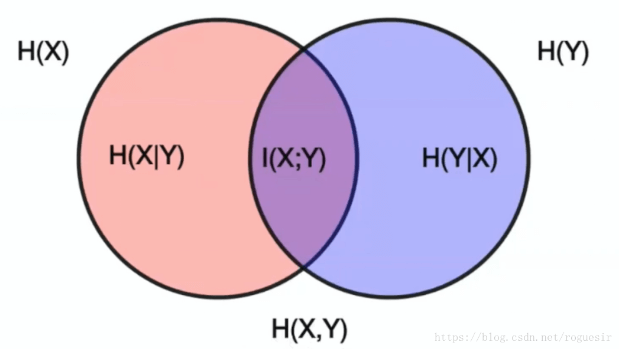## 信息熵#

$H(X) = - \sum_{x\in X}P(x)logP(x)$

## 条件熵#

$H(X|Y) = -\sum_{x\in X,y\in Y}P(x,y)logP(x|y)$

## 联合熵#

$H(X,Y) = -\sum_{i=1}^n \sum_{j=1}^n P(x_i,y_i)logP(x_i,y_i)$ $= \sum_{i=1}^n \sum_{j=1}^n P(x_i,y_i)log{1\over P(x_i,y_i )}$

## 信息增益#

$I(X;Y) = H(X) - H(X|Y)$

## 信息增益比（率）#

$g_R(D,A) = {g(D,A)\over H(D)}$## 基尼系数（Gini）#

$Gini(P) = \sum_{k=1}^K p_k(1-p_k) = 1 - \sum_{k=1}^K p_k^2$

## 相对熵（KL散度）#

$KL（P||Q）= \sum_{i=1}^n P(x_i)log{P(x_i)\over Q(x_i)}$

$KL(P||Q)\neq KL(Q||P)$

$JS(P||Q) = {1\over 2}[KL(P||Q) + KL(Q||P)]$

## 交叉熵（Cross-Entropy）#

$D_{KL}(P||Q) = \sum_{i=1}^n P(x_i)log(P(x_i)) - \sum_{i=1}^n P(x_i)log(Q(x_i))$ $= -H(P(x)) + [-\sum_{i=1}^n P(x_i)log(Q(x_i))]$

$H(P,Q) = -\sum_{i=1}^n P(x_i)log(Q(x_i))$

LR的损失函数就是交叉熵。

## 困惑度（Perplexity，PPL）#

$PP(S) = P(w_1w_2\dots w_n)^{-{1\over N}}$ $= \sqrt[N]{1\over{P(w_1w_2\dots w_n)}}$ $= \sqrt[N]{\prod_{i=1}^n {1\over P(w_i|w_1w_2\dots w_{i-1})}}$